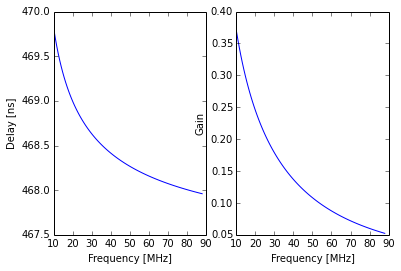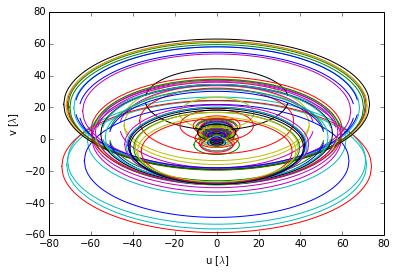# Basics¶

To obtain an ordered numpy array of stands connected to the DP system at a particular station:

In :
from lsl.common import stations

lwa1 = stations.lwa1
stands = lwa1.getStands()

print stands[:3]
print len(stands)

for i in xrange(5):
print str(stands[i])

[<lsl.common.stations.Stand object at 0x1101fb810>, <lsl.common.stations.Stand object at 0x1101fb810>, <lsl.common.stations.Stand object at 0x1101fb290>]
520
Stand 173:  x=-25.15 m, y=+22.99 m, z=+0.91 m
Stand 173:  x=-25.15 m, y=+22.99 m, z=+0.91 m
Stand 151:  x=-25.06 m, y=+47.76 m, z=+0.14 m
Stand 151:  x=-25.06 m, y=+47.76 m, z=+0.14 m
Stand 222:  x=-38.94 m, y=+14.20 m, z=+1.27 m

Although the stand list is useful, it is more useful to have a list of lsl.common.stations.Antenna instances. This provide much more information about the station setup (stand, FEE, position, etc.) than the simple stands list. To get this list:

In :
antennas = lwa1.getAntennas()

print antennas[:3]
print len(antennas)

for i in xrange(5):
print str(antennas[i])

[<lsl.common.stations.Antenna object at 0x11023ba90>, <lsl.common.stations.Antenna object at 0x11023bb50>, <lsl.common.stations.Antenna object at 0x110239910>]
520
Antenna 345: stand=173, polarization=0; digitizer 1; status is 3
Antenna 346: stand=173, polarization=1; digitizer 2; status is 3
Antenna 301: stand=151, polarization=0; digitizer 3; status is 3
Antenna 302: stand=151, polarization=1; digitizer 4; status is 3
Antenna 443: stand=222, polarization=0; digitizer 5; status is 3

## Stand Positions¶

Once you have an array of lsl.common.stations.Antenna instances you can do lots of things. For example, to get the location of stand #31 you can:

In :
for ant in antennas:
if ant.stand.id == 31 and ant.pol == 0:
print str(ant.stand)
print ant.stand.x, ant.stand.y, ant.stand.z

Stand 31:  x=-3.86 m, y=-21.79 m, z=+2.18 m
-3.856 -21.789 2.183

Or to get the distance between stand #31 and stand #45:

In :
import math

ant31 = None
ant45 = None
for ant in antennas:
if ant.stand.id == 31 and ant.pol == 0:
ant31 = ant
print str(ant.stand)
elif ant.stand.id == 45 and ant.pol == 0:
ant45 = ant
print str(ant.stand)

dx,dy,dz = ant31.stand-ant45.stand
print math.sqrt( dx**2 + dy**2 + dz**2 )

Stand 45:  x=-7.74 m, y=+21.11 m, z=+0.83 m
Stand 31:  x=-3.86 m, y=-21.79 m, z=+2.18 m
43.0976426966

In both of these examples, there is an extra "ant.pol == 0" in the "if" statement that selects the stands. This is needed because each stand has two dipole antennas, one for each polarization. If this extra requirement wasn't inlcuded, you'd have two matches:

In :
for ant in antennas:
if ant.stand.id == 31:
print str(ant.stand), ant.pol
print ant.stand.x, ant.stand.y, ant.stand.z

Stand 31:  x=-3.86 m, y=-21.79 m, z=+2.18 m 0
-3.856 -21.789 2.183
Stand 31:  x=-3.86 m, y=-21.79 m, z=+2.18 m 1
-3.856 -21.789 2.183

## Cable Information¶

lsl.common.statations.Antennas instance know more than just the stand location and name. They also store information about the cable that connects the antenna to the shelter and has methods to compute the cable delay and loss:

In :
%matplotlib inline
import numpy
from matplotlib import pyplot as plt

ant = antennas

print "Cable delay for '%s' @ 49 MHz: %.1f ns" % (ant.cable.id, ant.cable.delay(49e6)*1e9,)
print "Cable gain @ 49 MHz: %.4f" % ant.cable.gain(49e6)

freqs = numpy.linspace(10e6, 88e6, 101)
delays = ant.cable.delay(freqs)
gains = ant.cable.gain(freqs)

fig = plt.figure()
axD = fig.add_subplot(1, 2, 1)
axD.plot(freqs/1e6, delays*1e9)
axD.set_xlabel("Frequency [MHz]")
axD.set_ylabel("Delay [ns]")

axG = fig.add_subplot(1, 2, 2)
axG.plot(freqs/1e6, gains)
axG.set_xlabel("Frequency [MHz]")
axG.set_ylabel("Gain")
plt.show()

Cable delay for 'EXK-173-115 (Gray)' @ 49 MHz: 468.3 ns
Cable gain @ 49 MHz: 0.1106# Computing uv Tracks¶

lsl.common.statations.Antennas instance are also used by other LSL routines. For example, uv tracks can be computed with:

In :
from lsl.correlator import uvUtils

uv = uvUtils.computeUVTrack(antennas[:32:2])
print uv.shape

fig = plt.figure()
ax = fig.gca()
for i in xrange(uv.shape):
ax.plot(uv[i,0,:], uv[i,1,:])
ax.set_xlabel('u [$\\lambda$]')
ax.set_ylabel('v [$\\lambda$]')
plt.show()

(120, 2, 512)## Retrieving Earth Orientation Parameters¶

The lsl.misc.geodesy modules includes functions to retrieve earth orientation parameters (EOP; x, y, and UT1-UTC) for a given modified Julian Date (MJD) or MJD range. To retrieve the parameters as a lsl.misc.geodesy.EOP object, use:

In :
import time
from lsl import astro
from lsl.misc import geodesy

# Get EOPs for five days ago
jd = astro.unix_to_utcjd(time.time() - 3600*24*5)
mjd = jd - astro.MJD_OFFSET
eop = geodesy.getEOP(mjd)

# Report
print "MJD: %i" % eop.mjd
print "-> x: %.3f\"" % eop.x
print "-> y: %.3f\"" % eop.y
print "-> UT1-UTC Diff.: %.3f s" % eop.utDiff
print "-> Type: %s" % eop.type

MJD: 56564
-> x: 0.138"
-> y: 0.296"
-> UT1-UTC Diff.: 0.014 s
-> Type: final

Note: This may be slow/timeout depending on your connection.

For multiple MJDs, use lsl.misc.geodesy.getEOP() wiht a list to return a list of EOPs, one for each day:

In :
# Get EOPs for the last five days
jd1 = astro.unix_to_utcjd(time.time() - 3600*24*5)
jd2 = jd1 + 5
mjd1 = jd1 - astro.MJD_OFFSET
mjd2 = jd2 - astro.MJD_OFFSET
mjds = range(int(mjd1), int(mjd2)+1)
eops = geodesy.getEOP(mjds)

for eop in eops:
print "MJD: %i" % eop.mjd
print "-> x: %.3f\"" % eop.x
print "-> y: %.3f\"" % eop.y
print "-> UT1-UTC Diff.: %.3f s" % eop.utDiff
print "-> Type: %s" % eop.type

MJD: 56564
-> x: 0.138"
-> y: 0.296"
-> UT1-UTC Diff.: 0.014 s
-> Type: final
MJD: 56565
-> x: 0.135"
-> y: 0.295"
-> UT1-UTC Diff.: 0.013 s
-> Type: final
MJD: 56566
-> x: 0.133"
-> y: 0.294"
-> UT1-UTC Diff.: 0.012 s
-> Type: final
MJD: 56567
-> x: 0.131"
-> y: 0.293"
-> UT1-UTC Diff.: 0.011 s
-> Type: final
MJD: 56568
-> x: 0.129"
-> y: 0.292"
-> UT1-UTC Diff.: 0.010 s
-> Type: final
MJD: 56569
-> x: 0.128"
-> y: 0.291"
-> UT1-UTC Diff.: 0.009 s
-> Type: prediction

Note: This may be slow/timeout depending on your connection.